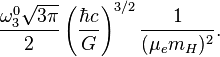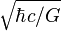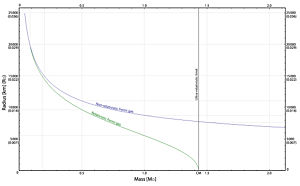# Chandrasekhar limit

The Chandrasekhar limit limits the mass of bodies made from electron-degenerate matter, a dense form of matter which consists of atomic nuclei immersed in a gas of electrons. The limit is the maximum nonrotating mass of an object that can be supported against gravitational collapse by electron degeneracy pressure. It is named after the astrophysicist Subrahmanyan Chandrasekhar, and is commonly given as being about 1.4 solar masses.

## Contents

As white dwarfs are composed of electron-degenerate matter, no nonrotating white dwarf can be heavier than the Chandrasekhar limit.

## Physics

As noted above, the Chandrasekhar limit is commonly given as being about 1.4 solar masses.

Stars produce energy through nuclear fusion, producing heavier elements from lighter ones. The heat generated from these reactions prevents gravitational collapse of the star. Over time, the star builds up a central core which consists of elements that the temperature at the center of the star is not sufficient to fuse. For main-sequence stars with a mass below approximately 8 solar masses, the mass of this core will remain below the Chandrasekhar limit, and they will eventually lose mass (as planetary nebulae) until only the core, which becomes a white dwarf, remains. Stars with higher mass will develop a degenerate core whose mass will grow until it exceeds the limit. At this point the star will explode in a core-collapse supernova, leaving behind either a neutron star or a black hole.

Computed values for the limit will vary depending on the approximations used, the nuclear composition of the mass, and the temperature. Chandrasekhar, eq. (36),, eq. (58),, eq. (43) gives a value of$\frac{\omega_3^0 \sqrt{3\pi}}{2}\left ( \frac{\hbar c}{G}\right )^{3/2}\frac{1}{(\mu_e m_H)^2}.$

Here, μe is the average molecular weight per electron, mH is the mass of the hydrogen atom, and ω30≈2.018236 is a constant connected with the solution to the Lane-Emden equation. Numerically, this value is approximately (2/μe)2 • 2.85 • 1030 kg, or 1.43 (2/μe)2 M, where M=1.989•1030 kg is the standard solar mass. As$\sqrt{\hbar c/G}$ is the Planck mass, MPl≈2.176•10−8 kg, the limit is of the order of MPl3/mH2.

Electron degeneracy pressure is a quantum-mechanical effect arising from the Pauli exclusion principle. Since electrons are fermions, no two electrons can be in the same state, so not all electrons can be in the minimum-energy level. Rather, electrons must occupy a band of energy levels. Compression of the electron gas increases the number of electrons in a given volume and raises the maximum energy level in the occupied band. Therefore, the energy of the electrons will increase upon compression, so pressure must be exerted on the electron gas to compress it. This is the origin of electron degeneracy pressure.Radius-mass relations for a model white dwarf. The green curve uses the general pressure law for an ideal Fermi gas, while the blue curve is for a non-relativistic ideal Fermi gas. The black line marks the ultra-relativistic limit.

In the nonrelativistic case, electron degeneracy pressure gives rise to an equation of state of the form P=K1ρ5/3. Solving the hydrostatic equation leads to a model white dwarf which is a polytrope of index 3/2 and therefore has radius inversely proportional to the cube root of its mass, and volume inversely proportional to its mass.

As the mass of a model white dwarf increases, the typical energies to which degeneracy pressure forces the electrons are no longer negligible relative to their rest masses. The velocities of the electrons approach the speed of light, and special relativity must be taken into account. In the strongly relativistic limit, we find that the equation of state takes the form P=K2ρ4/3. This will yield a polytrope of index 3, which will have a total mass, Mlimit say, depending only on K2.

For a fully relativistic treatment, the equation of state used will interpolate between the equations P=K1ρ5/3 for small ρ and P=K2ρ4/3 for large ρ. When this is done, the model radius still decreases with mass, but becomes zero at Mlimit. This is the Chandrasekhar limit. The curves of radius against mass for the non-relativistic and relativistic models are shown in the graph. They are colored blue and green, respectively. μe has been set equal to 2. Radius is measured in standard solar radii or kilometers, and mass in standard solar masses.

A more accurate value of the limit than that given by this simple model requires adjusting for various factors, including electrostatic interactions between the electrons and nuclei and effects caused by nonzero temperature. Lieb and Yau have given a rigorous derivation of the limit from a relativistic many-particle Schrödinger equation.

## History

In 1926, the British physicist Ralph H. Fowler observed that the relationship between the density, energy and temperature of white dwarfs could be explained by viewing them as a gas of nonrelativistic, non-interacting electrons and nuclei which obeyed Fermi-Dirac statistics. This Fermi gas model was then used by the British physicist E. C. Stoner in 1929 to calculate the relationship between the mass, radius, and density of white dwarfs, assuming them to be homogenous spheres. Wilhelm Anderson applied a relativistic correction to this model, giving rise to a maximum possible mass of approximately 1.37×1030 kg. In 1930, Stoner derived the internal energy-density equation of state for a Fermi gas, and was then able to treat the mass-radius relationship in a fully relativistic manner, giving a limiting mass of approximately (for μe=2.5) 2.19 • 1030 kg. Stoner went on to derive the pressure-density equation of state, which he published in 1932. These equations of state were also previously published by the Russian physicist Yakov Frenkel in 1928, together with some other remarks on the physics of degenerate matter. Frenkel's work, however, was ignored by the astronomical and astrophysical community.

A series of papers published between 1931 and 1935 had its beginning on a trip from India to England in 1930, where the Indian physicist Subrahmanyan Chandrasekhar worked on the calculation of the statistics of a degenerate Fermi gas. In these papers, Chandrasekhar solved the hydrostatic equation together with the nonrelativistic Fermi gas equation of state, and also treated the case of a relativistic Fermi gas, giving rise to the value of the limit shown above. Chandrasekhar reviews this work in his Nobel Prize lecture. This value was also computed in 1932 by the Soviet physicist Lev Davidovich Landau, who, however, did not apply it to white dwarfs.

Chandrasekhar's work on the limit aroused controversy, owing to the opposition of the British astrophysicist Arthur Stanley Eddington. Eddington was aware that the existence of black holes was theoretically possible, and also realized that the existence of the limit made their formation possible. However, he was unwilling to accept that this could happen. After a talk by Chandrasekhar on the limit in 1935, he replied:

The star has to go on radiating and radiating and contracting and contracting until, I suppose, it gets down to a few km. radius, when gravity becomes strong enough to hold in the radiation, and the star can at last find peace. … I think there should be a law of Nature to prevent a star from behaving in this absurd way!

Eddington's proposed solution to the perceived problem was to modify relativistic mechanics so as to make the law P=K1ρ5/3 universally applicable, even for large ρ. Although Bohr, Fowler, Pauli, and other physicists agreed with Chandrasekhar's analysis, at the time, owing to Eddington's status, they were unwilling to publicly support Chandrasekhar. Through the rest of his life, Eddington held to his position in his writings, including his work on his fundamental theory. The drama associated with this disagreement is one of the main themes of Empire of the Stars, Arthur I. Miller's biography of Chandrasekhar. In Miller's view:

Chandra's discovery might well have transformed and accelerated developments in both physics and astrophysics in the 1930s. Instead, Eddington's heavy-handed intervention lent weighty support to the conservative community astrophysicists, who steadfastly refused even to consider the idea that stars might collapse to nothing. As a result, Chandra's work was almost forgotten., p. 150

## Applications

The core of a star is kept from collapsing by the heat generated by the fusion of nuclei of lighter elements into heavier ones. At various points in a star's life, the nuclei required for this process will be exhausted, and the core will collapse, causing it to become denser and hotter. A critical situation arises when iron accumulates in the core, since iron nuclei are incapable of generating further energy through fusion. If the core becomes sufficiently dense, electron degeneracy pressure will play a significant part in stabilizing it against gravitational collapse.

If a main-sequence star is not too massive (less than approximately 8 solar masses), it will eventually shed enough mass to form a white dwarf having mass below the Chandrasekhar limit, which will consist of the former core of the star. For more massive stars, electron degeneracy pressure will not keep the iron core from collapsing to very great density, leading to formation of a neutron star, black hole, or, speculatively, a quark star. (For very massive, low-metallicity stars, it is also possible that instabilities will destroy the star completely.) During the collapse, neutrons are formed by the capture of electrons by protons, leading to the emission of neutrinos., pp. 1046–1047. The decrease in gravitational potential energy of the collapsing core releases a large amount of energy which is on the order of 1046 joules (100 foes.) Most of this energy is carried away by the emitted neutrinos. This process is believed to be responsible for supernovae of types Ib, Ic, and II.

Type Ia supernovae derive their energy from runaway fusion of the nuclei in the interior of a white dwarf. This fate may befall carbon-oxygen white dwarfs that accrete matter from a companion giant star, leading to a steadily increasing mass. It is believed that, as the white dwarf's mass approaches the Chandrasekhar limit, its central density increases, and, as a result of compressional heating, its temperature also increases. This results in an increasing rate of fusion reactions, eventually igniting a thermonuclear flame which causes the supernova., §5.1.2

Strong indications of the reliability of Chandrasekhar's formula are:

1. Only one white dwarf with a mass greater than Chandrasekhar's limit has ever been observed. (See below.)
2. The absolute magnitudes of supernovae of Type Ia are all approximately the same; at maximum luminosity, MV is approximately -19.3, with a standard deviation of no more than 0.3., (1) A 1-sigma interval therefore represents a factor of less than 2 in luminosity. This seems to indicate that all type Ia supernovae convert approximately the same amount of mass to energy.

## A type Ia supernova apparently from a supra-limit white dwarf

On April 2003, the Supernova Legacy Survey observed a type Ia supernova, designated SNLS-03D3bb, in a galaxy approximately 4 billion light years away. According to a group of astronomers at the University of Toronto and elsewhere, the observations of this supernova are best explained by assuming that it arose from a white dwarf which grew to twice the mass of the Sun before exploding. They believe that the star, dubbed the "Champagne Supernova" by David R. Branch, may have been spinning so fast that centrifugal force allowed it to exceed the limit. Alternatively, the supernova may have resulted from the merger of two white dwarfs, so that the limit was only violated momentarily. Nevertheless, they point out that this observation poses a challenge to the use of type Ia supernovae as standard candles.